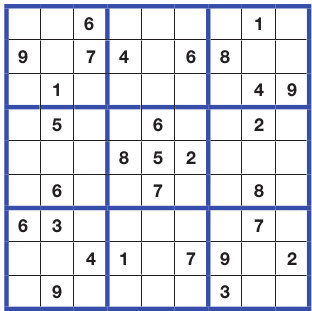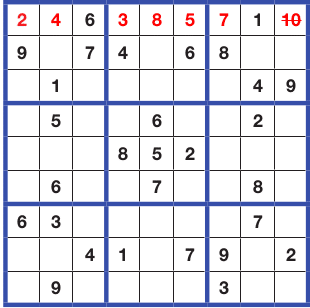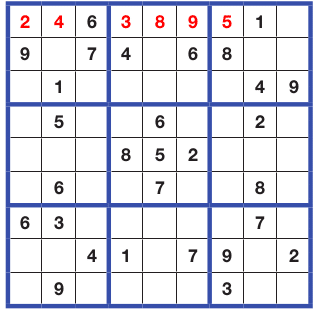# Solving Sudoku with a Simple Algorithm

2
6332Sudoku, a puzzle game, is a popular pastime and even an addiction with some people. Its origins date back to French newspapers of the 19th century. In its present form it has been popularised by a Japanese company, Nikoli, and has become mainstream since 1986. A simple backtracking algorithm can be used to solve a Sudoku puzzle.

The Sudoku puzzle has a 9×9 grid. The total grid area is divided into nine 3×3 sections. This grid has to be filled with digits in such a way that each 3×3 section contains all the digits from 1 to 9. At the same time, each row and each column of the 9×9 grid must also contain all the digits from 1 to 9 without repetition. Many hobbyists play the game on a regular basis. In the 9×9 grid, some digits are already filled in to make the permutation-combination possible. The remaining empty cells have to be filled. There have been many algorithms devised to solve Sudoku digitally in a PC, one of which is the brute force backtracking algorithm discussed in this article.

Algorithm overview

This backtracking algorithm is based on the depth-first principle of searching for a solution. It visits the first empty cell and fills it with the first possible digit. Then it moves forward until it finds a violation of the rule set. Once a violation is found, it goes back to the previously filled cell and tries to modify it. If there is still a violation it backtracks even more. Then it moves forward again until the puzzle is solved and all the cells are filled.

Let us try to solve the following Sudoku puzzle by using this algorithm.The total 9×9 grid is divided into nine 3×3 grids (bordered by the extra dark colour). Some of the cells are already filled. The algorithm has to be applied now.

The scan will start with the first blank position. Here, Row=0, Col=0 is the first blank square. It will look for the first allowed digit that can be filled in the corresponding 3×3 square. If we check the rows and columns in this 3×3 grid, the first number that is allowed is 2 (as 1 is in use). So 2 will be filled in the empty square. Then the scan will go for the next empty cell: Row=0, Col=1. Here, it will fill 4, as 3 is present in Col 1. Next comes Row=0, Col=3. This will be filled with 3. This goes on until Row=0, Col=8. According to our calculation, the next number must be 10, which is invalid. So we now have to backtrack and come to the previously edited cell, i.e., Row=0, Col=6, which currently has the number 7, as shown below.The numbers after 7 are 8 and 9. But both are not allowed in this cell, and we again reach the number 10 here. So now we have to backtrack to the previously edited cell, which is Row=0, Col=5. We can fill 9 there and move forward. Then we add the number 5 in Row=0, Col=6, as shown below. We go on this way until all the cells are filled up.This algorithm is simpler for beginners when compared to other algorithms. And as long as the puzzle is valid, a solution is guaranteed. However, it is slower than other algorithms.

Implementation overview (with code walk-through)

I have written code to implement the algorithm. (The full source code is given on GitHub at https://github.com/SupriyoGanguly/SudoSolver.git) Let’s take a quick look at the critical portion of the code.

• This program takes input of 9×9 numbers. Blank cells have to be filled with 100 while feeding input. Along with this it sets up a flag array, which will be used to implement backtrack and forward.
• Line 110: It will find the first blank position and store in p1.
```for(i=0;i<9;i++) { if(flag[r][i]==-1) {p1=i; break;} }

//check the first blank & hold the position in p1```
• get_notallowed returns an array not_allow filled with invalid digits. From this array, var will be derived, which is the valid digit for the blank cell at p1.
• Line 125: for(t=1;t<=9;t++) {if(var==t) {m=1; break;}}If var is not among 1 to 9, m=0 will be set. Then it will backtrack. Otherwise, m=1 will be set and a[r][p1]=var will be set.
• Inside if(m==0) it continuously backtracks using r1(row no.), p2(col no.) and finally sets a[r1][p2]=var=l at Line 146.
• flag[r][c]=0: Indicates the cell is pre-filled in input
• flag[r][c]=-1: Indicates the cell is empty in input
• flag[r][c]=-2: Indicates the cell has been filled by logic

Stochastic method and constraint programming are two other noted algorithms available for solving a Sudoku puzzle.

1.Mike McRoberts
2.Vinay Singh